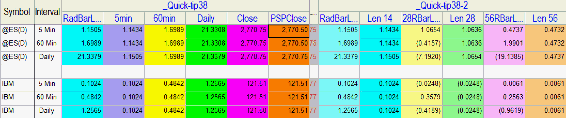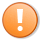# Quick-tip 38 | Plotting multiple period slopes on RadarScreen

Quick-tip 38 demonstrates how to calculate the slope for a price series on RadarScreen using the standard linear regression function. It then shows how to calculate and plot the slope for a 5 minute, 60 minute and daily price intervals on the same 5 min interval row.

A second program shows how to calculate the slope for different lengths using both the price of the closing price series of the row and a price series provider.### Video demonstration of Quick-tip 38This content is for members only.